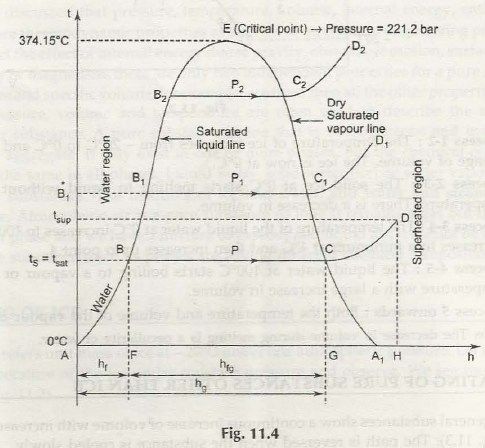In this case the water at A tends to have a temperature of 0°C and the different stages of it are explained as below

Induction stage- it shows the heating of water up to the boiling temperature. Sensible heat is defined  to raise the temperature of water(1 kg mass) from 0°C to boiling point . it is illustrated by  is denoted as hf” Sensible heat  = mass of water x specific heat x rise in temperature where

1 x 4.2 t s + 273- 273] = 4.2 l 5

Where specific heat of water is taken as 4.2 KJ / kg.

Warming stage- the change of the state from liquid to steam is illustrated by BC. FG is the heat absorbed during this stages and is illustrated by the latent heat of vaporization (h18).

Evaporation stage- the superheating process is shown by CD. During this stage the heat absorbed is GI,I which is known as the heat of superheat. The Line AH is a representation of the total heat that is required to go to the superheated point D

Effect of change on pressure

If the pressure bar is increased to P1, then the boiling temperature is also bound to increase.

point *B1 –the boiling temperature or saturating temperature at P1 bar.

C1- dry saturated steam

Line C1D1 shows the constant pressure process in which the steam is superheated (after C1 point).

In a similar stature, the family of curves is different for various situations. The line A- B-B1- B2 is referred to as the saturated liquid line, as it is the boundary between the water and the steam. On the other hand C2- CcC-A1, is known as dry saturated steam as it is known to formulate the boundary between the dry and the superheated steam. The pressure tends to increase the latent heat of vaporization, and this is the stage where the saturation temperature tends to decrease. It reaches a stage of  zero at E where the liquid and dry steam lines meet. At the point E is referred to as a critical point for sure. For steam, critical temperature is 374.15°C and critical pressure is 221.2 bar.

Total heat (enthalpy) of dry saturated steam (point C) is Jz8 = h1 + h/g”

where  hg = enthalpy of 1 kg of dry saturated steam

f = sensible heat of 1kg of water

fg = enthalpy of vaporisation of 1 kg of dry saturated steam = Latent heat of

steam.

The enthalpy of heating of water upto saturation temperature

f  = c pf (T sat – 273.16)

where              c  pf = 4.18 kj / kg k.

Wet steam

mg= mass of actual dry steam

m1= mass of water in suspension

m = mass of wet steam

Dryness fraction

x = mg / mg + mf

x is also called the quality of wet steam

for x = 0, it is saturated liquid

for x = 1, it is dry and saturated steam.

0 < x < 1.

Internal energy:     h = u + P f

f stands for fluid (water)

u1= hrPv1

for dry steam              u g = hg – P v g

g stands for gas (vapour).

(d) sp. enthalpy of wet steam:

h = h1+ xh fg

(e) sp. enthalpy of superheated steam:

sup = h f+ h fg + C pg U sup – t sat)

c g for steam = 2.1 KJ /kg0k.

t sup – t snt = Degree of superheat.

Specific volume: Specific volume of wet steam

v =v1+x(vg -v1)

= x vg + (1 – x) v1

Charles law when       P = C

sup / T sup = u g / T sat

(g) Specific entropy : specific entropy of wet steam

s = s1 + x (Sg – s1)

(h) Work done during evaporation

=P(vg -v1)

= Pv g as v1= negligible

(i) Work done during evaporation of a wet state

= Px vg.

(j) work done by superheated steam

‘Pv sup.

(k) specific entropy of steam:

The steam is produced at constant pressure. The entropy is assumed to be zero at 0°C.

Change m· spec1· f·1 c entropy= ds = dQ / T

(i) The heat supplied for heating 1 kg of water at constant pressure is given by

D θ = c pf dT

ds = c pf dT / T

Integrating

or                                 s = c pf in T sat / 273

(ii) The change in entropy during evaporation is given by

fg = h fg / T sat

(iii) The change in entropy during evaporation is given by

g = s f + s fg

= c pf in T sat / 273 + h fg / T sat

(iv) Entropy during superheating of steam is given by

(v) Entropy of superheated steam is given by

sup = s g + s gj

= c pf in T sat / 273 + h fg / T sat

wherecp g = sp heat of superheated steam

= 2.1 KJ/ kg° K

And                             c pf = 4.18 KJ/ kg0K.

Links of Next Mechanical Engineering Topics:-### Customer Reviews

My Homework Help
Rated 5.0 out of 5 based on 510 customer reviews at